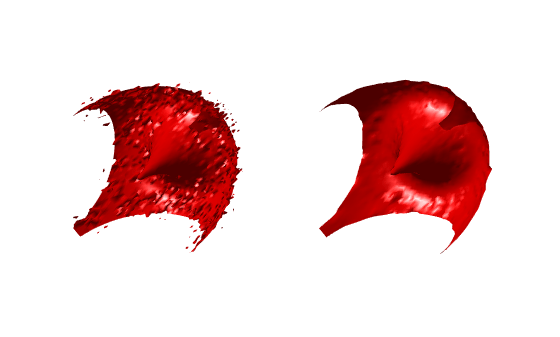# medfilt3

## 语法

``B = medfilt3(A)``
``B = medfilt3(A,[m n p])``
``B = medfilt3(___,padopt)``

## 说明

``B = medfilt3(A)` 使用 3×3×3 滤波器对三维图像 `A` 进行滤波。默认情况下，`medfilt3` 通过在边界处以镜像方式复制值来填充图像。`
``B = medfilt3(A,[m n p])` 对三维图像 `A` 执行三维中位数滤波。`B` 中的每个输出体素包含 `A` 中对应体素周围 m×n×p 邻域的中位数值。 `
``B = medfilt3(___,padopt)` 控制 `medfilt3` 如何填充数组边界。`

## 示例

```[x,y,z,V] = flow(50); noisyV = V + 0.1*double(rand(size(V))>0.95) - 0.1*double(rand(size(V))<0.05);```

`filteredV = medfilt3(noisyV);`

```subplot(1,2,1) hpatch1 = patch(isosurface(x,y,z,noisyV,0)); isonormals(x,y,z,noisyV,hpatch1) set(hpatch1,'FaceColor','red','EdgeColor','none') daspect([1,4,4]) view([-65,20]) axis tight off camlight left lighting phong subplot(1,2,2) hpatch2 = patch(isosurface(x,y,z,filteredV,0)); isonormals(x,y,z,filteredV,hpatch2) set(hpatch2,'FaceColor','red','EdgeColor','none') daspect([1,4,4]) view([-65,20]) axis tight off camlight left lighting phong```## 输入参数

`"symmetric"`用自身的镜像翻转填充数组
`"replicate"`通过重复边框元素填充数组
`"zeros"``0` 填充数组